# Debugging PHP applications with HHVM

In the previous parts of this series we got you started with HHVM and showed how we could get the symfony standard edition running on HHVM. This time we will dive deeper into HHVM by using it to debug our application.

For most people the easiest way of debugging a PHP application is to place `var_dump()` and `die()` statements all over the code. Another option is installing xdebug, which has gotten a lot easier nowadays due to IDE integrations.

In this blog post we'll show you how to debug your PHP application using HHVM. We describe how you can step through your program, set and manage your breakpoints, how to inspect variables and take a peek at helpful features like conditional breakpoints.

Note: for all examples in this post we use the HHVM 2.2.0 precompiled binaries installed on a vagrant box running Ubuntu 12.04 (`apt-get` installable!).

## A faulty program

Let's start off by creating a "faulty" program we can debug. Create a simple script and save it as `example.php`:

``````<?php
\$a = 4; \$b = 2;
echo divide(\$a, \$b) . "\n";
\$a = 5; \$b = 0;
echo divide(\$a, \$b) . "\n";

function divide(\$a, \$b)
{
return \$a / \$b;
}
``````

Running this example with `hhvm example.php` prints a warning. We will try to find the bug using the hhvm debugger.

## Firing up the debugger

``````hhvm --mode debug example.php
``````

HHVM starts and loads the program, but does not execute it yet. HHVM is waiting for a command. Run the example by giving the `run` command.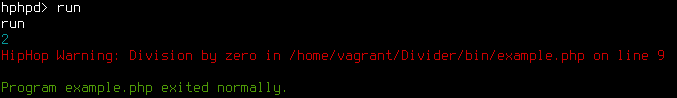## Stepping through the program

A first approach to debugging this program would be to walk through each step of the execution. With `next` we can do exactly that. This time start the program using the `continue` command. This loads our program and pauses it just before the first line of code. Use the `next` command to execute the program one line at the time.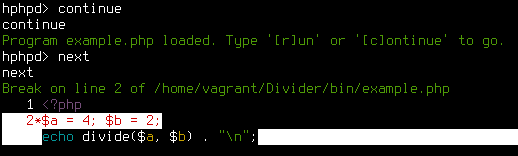You may have noticed that the debugger did not step into the divide() function. To check out what is going on in divide(), we can use the `step` command as soon as we reach line 3. `step` lets us step into a function.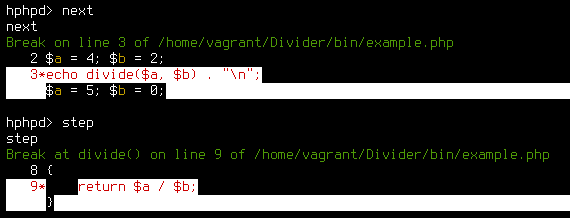The counterpart of `step` is the `out` command. It can be used to continue running the program until it gets to the point where the function was called.

## Our first breakpoint

Stepping through an entire program by hand is a bit cumbersome. In fact we want HHVM to pause the script when it enters the divide() function. This can be done by setting a breakpoint. A breakpoint is an intentional stop or pause placed in a program. In HHVM we set a breakpoint with the `break` command.

``````break divide() # break when the divide function is called
``````

This will set a new breakpoint when HHVM enters the divide function. Start the program again with the `run` command. HHVM now pauses when it hits the breakpoint.To continue the execution give the `continue` command. The program resumes execution and breaks again when the breakpoint is hit a second time. `continue` the execution. Now the program gives the warning and finally it ends normally.

Other possibilities for setting breakpoints include:

``````break example.php:9 # break on line 9 of example.php
break Math::divide() # break when the divide function of the Math class is called
``````

## Inspecting variables

When our program hits a breakpoint and pauses execution, we can inspect the value of all variables in the current scope by using the `print` command. Run the program again (`run`). When it breaks, inspect the value of `\$a` and `\$b` with the following commands.

``````print \$a
print \$b
``````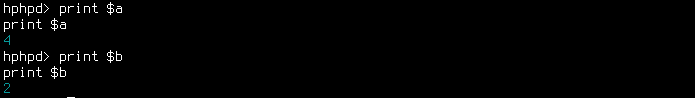Continue (`continue`) the program and inspect `\$a` and `\$b` on the second break. We will notice `\$b` has a value of `0`. When we divide a number by `0`, we will get a "Division by zero" warning.

## Conditional breakpoints

Now we know what causes the problem, we have to find out where `\$b` is set to `0`. First we need to execute the program until it enters the divide function with `\$b` being `0`. One approach is to inspect `\$b` every time it enters divide().

In this case divide() is called only two times, but imagine a situation where this function is called 1337 times. You definitely don't want to inspect and continue that many times to find a situation where `\$b` is equal to `0`. Conditional breakpoints to the rescue!

A conditional breakpoint only breaks the program when a certain condition is met. The syntax in hhvm is `break <location> if <condition>`.

In our case we're interested in the situation where `\$b` is equal to `0`. Start of by clearing the previously set breakpoints using:

``````break clear all
``````

Then set a conditional breakpoint with:

``````break divide() if \$b == 0
``````

Run the program and notice that HHVM doesn't break until `\$b` is equal to `0`. Continue to see the program error out again.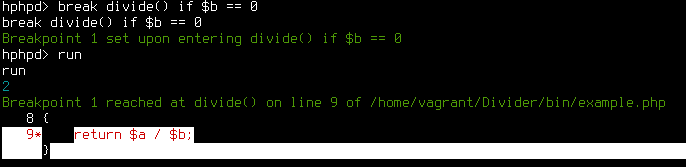## Getting a trace

Now we have found a point in our program where `\$b` is equal to `0`, we want to know from where divide() was called, so we can take a look at that piece of code to find out the reason why `\$b` is equal to `0`.

HHVM gives you a stack trace of the current breakpoint when you use the `where` command. Run the program again, and use `where` as the debugger breaks the program execution.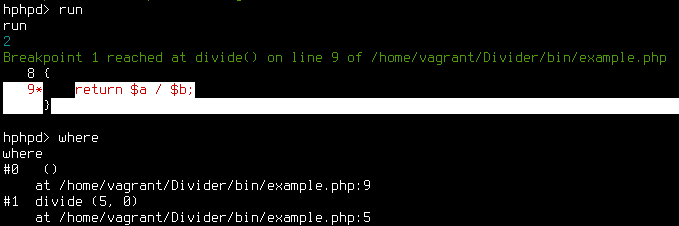## Managing breakpoints

If we place a lot of breakpoints we can lose track of all the breakpoints we have set. HHVM provides a list of all breakpoints with the `break list` command.

All breakpoints are given a number (e.g. 1), making it possible to remove a breakpoint (`break clear 1`) or temporarily disable one or all breakpoints with the commands:

``````break disable 1
break enable 1
break disable all
break enable all
``````

For a complete overview of all the possibilities use the `break help` command.

## Wrap up

In this post we've introduced you to debugging with HHVM. In the next blog post we'll move from debugging a cli program to debugging web requests!

The HHVM debugger has been quite solid for us so far. The only improvement we could come up for now would be vim integration!

#### » We're hiring! «

Come and join us in our quest to build the best development team in the universe...and beyond!